# Solution assignment 06 Linear functions and graphs

### Assignment 6

The linethrough the point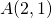with slope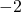intersects the line: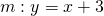in point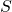.
Calculate the coordinates of.

### Solution

First we determine the line. We use the formula: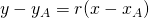in which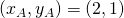are the coordinates of the point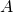and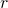is the slope of, so. We find for: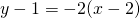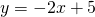In order to calculate the coordinates of, we solve (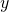-values of the intersection pointare equal):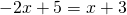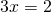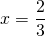and find the corresponding-coordinate: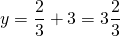The result can seen in the figure.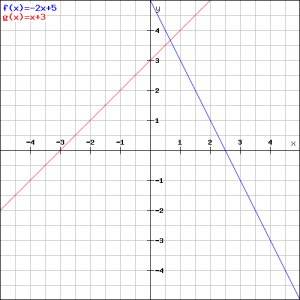Identify which line is which.